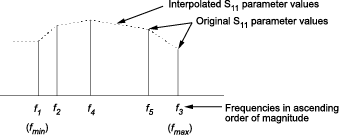# rfckt.datafile

Component or network from file data

## Description

Use the `datafile` object to represent RF components and networks that are characterized by measured or simulated data in a file.

Use the `read` method to read the data from a file in one of the following formats:

• Touchstone

• Agilent® P2D

• Agilent S2D

• AMP

## Creation

### Syntax

``h = rfckt.datafile``
``h = rfckt.datafile(Name,Value)``

### Description

example

````h = rfckt.datafile` returns a circuit object whose properties all have their default values.```
````h = rfckt.datafile(Name,Value)` sets properties using one or more name-value pairs. For example, `rfckt.datafile('IntType','Cubic')` creates an RF component or network that uses cubic interpolation. You can specify multiple name-value pairs. Enclose each property name in a quote. Properties not specified retain their default values.```

## Properties

expand all

This property is read-only.

Computed S-parameters, noise figure, OIP3, and group delay values, specified as a `rfdata.data `object. For more information refer, Algorithms.

Data Types: `function_handle`

File name containing circuit data, specified as a `1-by-1` character array.

Data Types: `char`

Interpolation method, specified as a `1-by-N` character array of the following values:

MethodDescription
`Linear` (default)Linear interpolation
`Spline`Cubic spline interpolation
`Cubic`Piecewise cubic Hermite interpolation

Data Types: `char`

This property is read-only.

Object name, specified as a `1-by-N` character array.

Data Types: `char`

This property is read-only.

Number of ports, specified as a positive integer. The default value is 2.

Data Types: `double`

## Object Functions

 `analyze` Analyze RFCKT object in frequency domain `calculate` Calculate specified parameters for rfckt objects or rfdata objects `circle` Draw circles on Smith Chart `extract` Extract specified network parameters from rfckt object or data object `listformat` List valid formats for specified circuit object parameter `listparam` List valid parameters for specified circuit object `loglog` Plot specified circuit object parameters using log-log scale `plot` Plot circuit object parameters on X-Y plane `plotyy` Plot parameters of RF circuit or RF data on X-Y plane with two Y-axes `getop` Display operating conditions `polar` Plot specified object parameters on polar coordinates `semilogx` Plot RF circuit object parameters using log scale for x-axis `semilogy` Plot RF circuit object parameters using log scale for y-axis `smith` Plot circuit object parameters on Smith chart `write` Write RF data from circuit or data object to file `getz0` Calculate characteristic impedance of RFCKT transmission line object `read` Read RF data from file to new or existing circuit or data object `restore` Restore data to original frequencies `getop` Display operating conditions `groupdelay` Group delay of S-parameter object or RF filter object or RF Toolbox circuit object

## Examples

collapse all

Represent RF components and networks that are characterized by measured or simulated data in a file using rfckt.datafile.

`data=rfckt.datafile('File','default.s2p')`
```data = rfckt.datafile with properties: IntpType: 'Linear' File: 'default.s2p' nPort: 2 AnalyzedResult: [1x1 rfdata.data] Name: 'Data File' ```

## Algorithms

The `analyze` method computes the `AnalyzedResult` property using the data stored in the `File` object property. If the file you specify with this property contains network Y- or Z-parameters, `analyze` first converts these parameters, as they exist in the `rfckt.datafile` object, to S-parameters. Using the interpolation method you specify with the `'IntpType'` property, `analyze` interpolates the S-parameters to determine the S-parameters at the specified frequencies. Specifically, `analyze` orders the S-parameters according to the ascending order of their frequencies, fn. It then interpolates the S-parameters, using the MATLAB® `interp1` function. For example, the curve in the following diagram illustrates the result of interpolating the S11 parameters at five different frequencies.For more information, see “One-Dimensional Interpolation” and the `interp1` reference page in the MATLAB documentation.

 EIA/IBIS Open Forum, Touchstone File Format Specification, Rev. 1.1, 2002